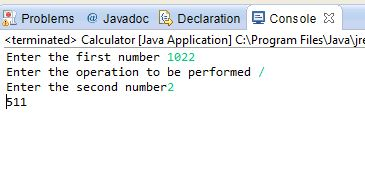# Conditional Statements in JAVA

## Conditional Statements in JAVA

In this tutorial you will learn: 1. JAVA coding essentials 2. Taking input in java console 3. JAVA code for making a calculator 4. How the basic arithmetic operations are performed in JAVA Making Java Project Basic Java console program: • Open Eclipse > Workspace • File > new > java project • File > new > class Basic Coding Strategy for making a Calculator In this tutorial I will teach you guys how to make a calculator, the calculator here can perform the operations of addition, subtraction, multiplication and division. A good coder always makes a concrete plan and strategy before starting to write his code. The structure will be as, first we will take input of two numbers by the user, take in the operation as input and will perform the operation and display the result back to the user. Here for each of the operation we will use a separate portion of the program. Java programming is totally object oriented based, and everything here is done in classes, so here we have first declared the main(which is also inside the class) of the program here in which we are first taking the inputs by the user, as you can clearly see that for the first number ,by using the scanner class object we get the number and store it in the variable ‘num1’, then same procedure is followed for the second integer which is ‘num2’ and also for the symbol declared as ‘choice’. Here the data types must be carefully determined.
` import java.util.Scanner; public class Calculator{ public static void main(String[] args) {int num1,num2;char choice;} `
In this part of the code we are taking the inputs from the user and storing it in the variables ‘num1’,’num2’ and ‘choice’ which we declared in the above code. ‘System.out.print’ command is used here to display whatever it is given input on the screen and we are using the input scanner object to store the data into the variables. It must be noted here that the syntax for the char data type is different, it is because the scanner object is specifically made for the int as can be seen in the code below.
`System.out.print("Enter the first number ");Scanner inputScanner= new Scanner(System.in);num1 = inputScanner.nextInt();  System.out.print("Enter the operation to be performed ");choice = inputScanner.next().charAt(0);//1 for addition  System.out.print("Enter the second number");                               num2 = inputScanner.nextInt();`
In this part of the code I am using the if statements to see the choice of the user that whether he chooses addition, subtraction, multiplication or division and accordingly the operation is performed and displayed to the user. Considering the first if statement “if(choice =='+')” , this statement checks the value stored in variable ‘choice’ and if it is ‘+’ the statement will do addition , similarly for the other conditions we perform a different operations by checking the character stored in the variable choice.
` if(choice =='+'){System.out.println(num1+num2); //add}else if(choice=='-'){System.out.println(num1-num2); //subtract}else if(choice=='*'){System.out.println(num1*num2); //multiply} else  if(choice=='/'){System.out.println(num1/num2); //divide}}} `
Note: The name of your public class should be the same as your class name. In this tutorial I have taught you guys to make a calculator by the simplest way possible which is using if/else statement, same result can be achieved by using switch statements. We can also use functions for the purpose , keep following they will be taught in upcoming tutorials.We will learn more coding from next tutorials. If you have any questions or want to learn programming from me simply add me on skype Username: mkprogrammer2121